# LCD Display Inverter

Display Inverter / VGA Board / LCD Controller

## Thermal Management Calculations for RF Amplifiers in LFCSP and Flange Packages

Radio frequency (RF) amplifiers are available in lead frame chip scale packages (LFCSPs) and flanged packages, and are mounted on printed circuit boards (PCBs) through proven reflow soldering processes. Not only does the PCB serve as an electrical interconnection between components, it is also the main way for the amplifier to dissipate heat (using the metal bumps on the bottom of the package).

By Eamon Nash Analog Devices

Introduction

Radio frequency (RF) amplifiers are available in lead frame chip scale packages (LFCSPs) and flanged packages, and are mounted on printed circuit boards (PCBs) through proven reflow soldering processes. Not only does the PCB serve as an electrical interconnection between components, it is also the main way for the amplifier to dissipate heat (using the metal bumps on the bottom of the package).

This application note introduces the concept of thermal resistance and provides a technique for modeling the flow of heat from the die to the heat sink of a typical RF amplifier in an LFCSP or flange package.

Hot Concept Review

heat flow

When there is a temperature difference between different areas of the material, heat flows from the high temperature area to the low temperature area. This process is similar to electric current, which flows through a circuit from a high-potential region to a low-potential region.

Thermal resistance

All materials have some thermal conductivity. Thermal conductivity is a measure of a material’s ability to conduct heat. Thermal conductivity values ​​are usually given in watts per meter kelvin (W/mK) or watts per inch kelvin (W/inK). If the thermal conductivity of the material is known, the following formula is used to calculate the thermal resistance (θ) per unit volume of the material in C/W or K/W: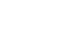(1)

in:

Length indicates the length or thickness of the material in meters.

k is the thermal conductivity of the material.

Area represents the cross-sectional area, in m2for the unit.

temperature

Using the analogy that heat flow is equivalent to the amount of electric current, the temperature difference of a material that inherently has thermal resistance and supports heat flow is as follows:

ΔT = Q × θ (2)

in:

ΔT represents the temperature difference (K or °C) between different regions of the material.

Q represents heat flow (W).

θ represents the thermal resistance (C/W or K/W) of the material.

device thermal resistance

The thermal resistance of a device is quite complex and tends to be nonlinear with temperature. Therefore, we use the finite element analysis method to establish the thermal model of the device. Infrared photography can determine the temperature at the device connections and the temperature of the package during operation. Based on these analyses and measurements, the equivalent thermal resistance can be determined. Equivalent thermal resistance is valid under the specific conditions under which the device is measured, typically at the maximum operating temperature.

Refer to Table 1 for a table of absolute maximum ratings for typical RF amplifiers.

Table 1. Absolute Maximum Ratings for Typical RF Amplifiers

 parameter Rated value Drain Bias Voltage (VDD) 60Vdc Gate Bias Voltage (VGG1) -8 V to 0 V dc Radio Frequency (RF) Input Power (RFIN) 35dBm Continuous power consumption (PDISS) (T = 85°C) (636 mW/°C derated above 85°C) 89.4W Thermal Resistance, Junction to Pad Backside (θJC) 1.57°C/W temperature range storage -55°C to +150°C Operating temperature -40°C to +85°C Junction temperature range (TJ) 225°C Nominal Junction Temperature (TCASE = 85°C, VDD = 50 V) 187°C

For LFCSP and flange packages, the package case is assumed to be the metal block on the bottom of the package.

Maximum junction temperature

In a given data sheet, the maximum junction temperature (based on the device’s semiconductor process) is given in the Absolute Maximum Ratings table for each product. In Table 1, the specified maximum junction temperature for the million-hour MTTF is 225°C. This temperature specified is generally applicable to Gallium Nitride (GaN) devices. Exceeding this limit can result in reduced device life and permanent device failure.

range of working temperature

device operating temperature (TCASE) are given on the package base. TCASEis the temperature of the metal bump on the bottom of the package. The operating temperature is not the temperature of the air surrounding the device.

If T is knownCASEand PDISS, it is easy to calculate the junction temperature (TJ). For example, if TCASE=75°C, PDISS=70 W, then T can be calculated using the following formulaJ:

TJ = TCASE + (θJC × PDISS)
= 75°C + (1.57°C/W × 70W)
= 184.9°C

When considering device reliability, TJis the most important specification parameter and should never be exceeded. Conversely, if it is possible to reduce PDISS, so that TJremains below the maximum allowable level, the TCASESpecified Absolute Maximum Ratings may be exceeded. In this example, when the case temperature exceeds the specified maximum value of 85°C, a derating value of 636 mW/°C can be used to calculate the maximum allowable PDISS. For example, using the data in Table 1, when PDISSWhen the limit is 83 W, the maximum allowable TCASEis 95°C. PDISSIt can be calculated using the following formula:

PDISS = 89.4 W − (636 mW/°C × 10°C)
= 83W

use this PDISS value, the junction temperature of 225°C can be calculated using the following formula:

TJ = TCASE + (θJC × PDISS)
= 95°C + (1.57°C/W × 83 W) (3)

Thermal model of device and PCB environment

To fully understand the entire thermal environment around a device, the heat dissipation paths and materials of the device must be modeled. Figure 1 shows a cross-sectional schematic of the LFCSP package mounted on the PCB and heat sink. In this example, the die generates heat, which is then transferred to the heat sink via the package and PCB. To determine the temperature at the device junction, thermal resistance must be calculated. Using thermal resistance and heat flow, the junction temperature can be calculated. The junction temperature is then compared to the maximum specified junction temperature to determine if the device is operating reliably.

In Figure 1, the thermal path from the device connection to the heat sink is defined as follows:

• θJAis the thermal resistance from the device connection to the air around the top of the package.

• θJCis the thermal resistance from the connection to the case (the metal block on the bottom of the package).

• θSN63is the thermal resistance of the solder.

• θCUis the thermal resistance of the copper plating on the PCB.

• θVIACUis the thermal resistance of the copper plating on the through hole.

• θVIASN63is the thermal resistance of the solder filled in the via.

• θPCBis the thermal resistance of the PCB laminate.

In a typical circuit board, there are multiple vias and multiple PCB layers. When calculating the thermal resistance of the cross section of the system, the thermal circuit is used to calculate the individual thermal resistances, and the series thermal resistance is combined with the parallel thermal resistance to determine the total thermal resistance of the device.Figure 1. Thermal model of LFCSP package mounted on PCB and heat sink

System thermal resistance calculation

For each heat dissipation path, use Equation 1 to calculate its thermal resistance. To calculate the individual thermal resistance values, the thermal conductivity of the material must be known. See Table 2 for thermal conductivity of commonly used materials in PCB assemblies.

Table 2. Thermal Conductivity of Commonly Used PCB Materials

 Material Thermal conductivity (W/inK) Copper (Cu) 10.008 Aluminum (Al) 5.499 Rogers 4350 (RO4350) 0.016 FR4 or G-10 laminate 0.008 Alumina (Al2O3) 0.701 SN63 Solder 1.270 Thermally Conductive Epoxy 0.020 Gallium Arsenide (GaAs) 1.501 molding compound 0.019

Figure 2 shows the equivalent thermal circuit based on the thermal model shown in Figure 1. TPKGIndicates the temperature at the bottom of the package, TSINKIndicates the temperature of the radiator. In Figure 2, it is assumed that the package (TA) the ambient air temperature is constant. For real assemblies with outer shells, TAMay rise as power consumption increases. The thermal path to ambient air temperature is ignored for this analysis because for LFCSP and flange packages with metal blocks, θJAmuch larger than θJC.Figure 2. Equivalent thermal circuit

Thermal Resistance Example: HMC408LP3 Evaluation Board

The HMC408LP3 power amplifier uses a 0.01-inch thick evaluation board constructed of Rogers RO4350 laminate. The ground pad shown in Figure 3 has an area of ​​0.065 × 0.065 inches with five 0.012-inch diameter vias. The top and bottom of the board each have 1 oz copper plating (0.0014″ thick). The vias are plated with ½ oz copper (0.0007″ thick). During assembly, the through holes are filled with SN63 solder. Analysis showed that almost all of the heat flow would flow through the solder-filled vias. Therefore, the rest of the board layout can be ignored in this analysis.Each thermal resistance is calculated using Equation 1. Calculate thetaSN63, the thermal conductivity of the SN63 solder used is 1.27 W/inK, the length (or thickness of the solder joint) is 0.002 inches, and the solder area is 0.004225 inches (0.065 inches × 0.065 inches).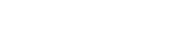(4)

Next, calculate the value of the copper plating on top of the PCB in a similar manner. The thermal conductivity of the copper coating is 10.008 W/inK, the length is 0.0014 inches (1 oz copper), and the coating area is 0.00366 square inches (in2).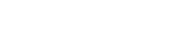(5)

For the area of ​​copper plating on the through hole, use the following formula to calculate

Area = π × (rO2 C rI2) (6)

in:

rOIndicates the outer diameter.

rIIndicates the inner diameter.

With an outside diameter of 0.006 inches and an inside diameter of 0.0053 inches, the calculated area is 0.00002485 in2. The length of the vias is the thickness of the board (0.01 inches), and the thermal conductivity of copper is 10.008 W/inK.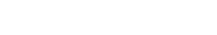(7)

Since there are 5 vias side by side, the thermal resistance is divided by 5. So, θVIACU = 8.05°C/W.

In a similar manner, the value of the fill solder for the vias is calculated.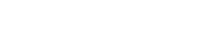(8)

Since there are 5 filled vias, the equivalent thermal resistance is θVIASN63 = 17.85°C/W.

Next, use a Rogers RO4350 thermal conductivity of 0.01 inch length, 0.016 W/inK, and 0.00366 in2Area calculates the thermal resistance of the PCB.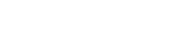(9)

In the equivalent thermal circuit shown in Figure 2, the three thermal resistances (θPCB, θVIACUand θVIASN63) is 5.37°C/W after the parallel combination. After filling the vias with solder, the thermal resistance decreased from 8.05°C/W to 5.37°C/W. Finally, adding the value of the thermal resistance in series gives the thermal resistance of the entire PCB assembly.

θASSY = thetaSN63 + thetaCU + thetaEQUIV + thetaCU = 0.372 + 0.038 + 5.37 + 0.038 = 5.81°C/W (10)

where θASSYIndicates the thermal resistance of the assembly.

Determine power consumption

After the thermal resistance value is determined, the heat flow (Q) value must be determined. For RF devices, the value of Q represents the difference between the total power input to the device and the total power output from the device. Total power includes RF power and DC power.

Q = PINTOTAL − POUTTOTAL = (PINRF +PINDC) − POUTRF (11)

in:

PINTOTALIndicates the sum of DC power and RF input power.

POUTTOTALrepresents the power output by the device, and POUTRFsame.

PINRFIndicates RF input power.

PINDCIndicates DC input power.

POUTRFIndicates the RF output power delivered to the load.Figure 4. HMC408LP3 power dissipation vs input power

For the HMC408LP3 power amplifier, use Equation 11 to calculate P shown in Figure 4DISSvalue of . Figure 4 shows the following characteristics of the amplifier:

• The device consumes approximately 4 W with no RF input signal.

• When using RF signals, PDISSThe value of is determined by the frequency.

• At a certain input power, the device consumes the lowest power dissipation.

According to the equivalent thermal resistance, θTOTALand Q, the junction temperature can be calculated using

ΔT = Q × θTOTAL (12)

θTOTAL = thetaASSY + thetaJC = 5.81 + 13.79 = 19.6°C/W (13)

For quiescent state with no RF input power, Q = 4 W, and

ΔT = 4.0 × 19.6 = 78.4°C (14)

Because the maximum junction temperature of the HMC408LP3 is specified at 150°C, at PDISS = 4 W, the temperature of the heat sink must be ≤71.6°C (that is, 78.4°C + 71.6°C = 150°C).

The HMC408LP3 power amplifier consumes less than 4 W during normal operation (eg, input power ≤ 5 dBm), which means that the heat sink temperature can be slightly higher than 71.6°C. However, if the amplifier is operating in a deep compression environment and the input power is equivalent to 15 dBm, then PDISSincreases and requires the heat sink temperature to be below 71.6°C.

Table 3. Thermal Operating Data Sheet

 describe value unit Notes Heatsink maximum temperature 70 °C θASSY 5.81 °C/W Calculated from an equivalent thermal circuit θJC 13.79 °C/W from datasheet θTOTAL 19.6 °C/W add thetaASSYand θJC Q 4.0 W The resulting junction temperature 148.4 °C Heatsink maximum temperature + (θTOTAL × Q); do not exceed the maximum channel temperature listed in the data sheet

reliability

The life expectancy of a component is closely related to the operating temperature. Operating at temperatures below the maximum junction temperature can extend the life of the device. Exceeding the maximum junction temperature will shorten the lifetime. Therefore, performing a thermal analysis ensures that the specified maximum junction temperature will not be exceeded under expected operating conditions.

in conclusion

Using low junction temperature surface mount RF power amplifiers in LFCSP and flange packages to enclose thermal resistance forces the PCB not only to act as an RF interconnect between devices, but also as a thermal path to conduct heat away from the power amplifier.

Therefore, θJC replace thetaJA, which has become an important thermal resistance index to measure the LFCSP or flange package.

In these calculations, the most critical metric is the RF amplifier’s junction or channel temperature (TJ). As long as the maximum junction temperature is not exceeded, then other nominal limits such as TCASE, it can be higher than the limit.